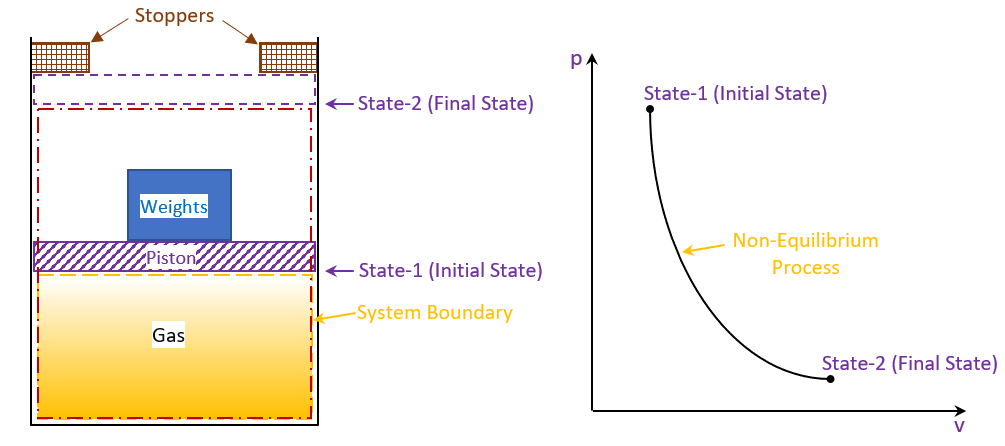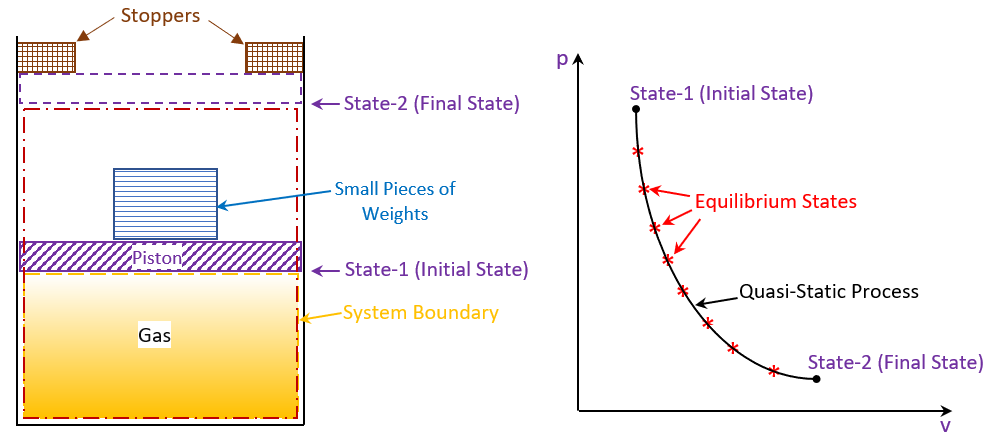### Quasistatic Process

Quasistatic ProcessFigure 1: Non-Equilibrium Process

Let us consider a system as in figure 1. The system initially is in equilibrium state represented by the state 1 with properties p1 and V1. The weight on the piston just balances upward force exerted by the gas. If the weight is removed there will be an unbalanced force between the system and the surroundings. Due to gas under pressure the piston will move up till it hits the stoppers. The system again comes to equilibrium state being described by the state 2 with properties p2 and V2. But the intermediate states passed through by the system are non-equilibrium states, which cannot be described by thermodynamic coordinates as in figure 1. In figure 1 state 1 and 2 are the initial and final equilibrium states joined by a line which has got no meaning otherwise.

Now if the single weight on the piston is made up of many very small pieces of weights as in figure 2. And these weights are removed one by one very slowly from the top of the piston at any instant of the upward travel of the piston and if the gas system is isolated, the departure of the state of the system from the thermodynamic equilibrium state will be infinitesimally small. So every state passed through by the system will be an equilibrium state. Such a process which is but a locus of all the equilibrium points passed through by the system is known as “Quasi-Static” process as in figure 2Figure 2: Quasi-Static Process

“Quasi’’ meaning “Almost” - Infinite slowness is the characteristic feature of a quasi static process. A quasi static process is thus the succession of an equilibrium state. A quasi-static process is also called a “Reversible Process”.